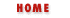#Chapter 4Expressions and Operators## 4.7 Bitwise Operators

Despite the fact that all numbers in JavaScript are floating-point, the bitwise operators require numeric operands that have integral values. They operate on these integer operands using a 32-bit integer representation instead of the equivalent floating-point representation. These operators may return NaN if used with operands which are not integers or which are too large to fit in a 32-bit integer representation. Four of these operators perform boolean algebra on the individual bits of the operands, behaving as if each bit in each operand was a boolean value and performing similar operands to the logical operators we saw earlier. The other three bitwise operators are used to shift bits left and right.

If you are not familiar with binary numbers and the binary representation of decimal integers, you can skip the operators described in this section. The purpose of these operators is not described here; they are used for low-level manipulation of binary numbers and are not commonly used in JavaScript programming.

### Bitwise And (&)

The & operator performs a boolean AND operation on each bit of its integer arguments. A bit is set in the result only if the corresponding bit is set in both operands.

### Bitwise Or (|)

The | operator performs a boolean OR operation on each bit of its integer arguments. A bit is set in the result if the corresponding bit is set in one or both of the operands.

### Bitwise Xor (^)

The ^ operator performs a boolean "exclusive OR" operation on each bit of its integer argument. Exclusive OR means either operand one is true or operand two is true, but not both. A bit is set in the result of this operation if a corresponding bit is set in one (but not both) of the two operands.

### Bitwise Not (~)

The ~ operator is a unary operator that appears before its single integer argument. It operates by reversing all bits in the operand. Because of how signed integers are represented in JavaScript, applying the ~ operator to a value is equivalent to changing its sign and subtracting 1.

### Shift Left (<<)

The << operator moves all bits in its first operand to the left by the number of places specified in the second operand, which should be an integer between 1 and 31. For example, in the operation a << 1, the first bit (the ones bit) of a becomes the second bit (the twos bit), the second bit of a becomes the third, etc. A zero is used for the new first bit, and the value of the 32nd bit is lost. Shifting a value left by one position is equivalent to multiplying by 2. Shifting two positions is equivalent to multiplying by 4, and so on.

### Shift Right with Sign (>>)

The >> operator moves all bits in its first operand to the right by the number of places specified in the second operand (an integer between 1 and 31). Bits that are shifted off the right are lost. The bits filled in on the left are the same as the sign bit of the original operand to preserve the sign of the result: If the first operand is positive, the result will have zeros filled in the high bits; if the first operand is negative, the result will have ones filled in the high bits. Shifting a value right one place is equivalent to dividing by two (discarding the remainder), shifting right two places is equivalent to integer division by four, and so on.

### Shift Right Zero Fill (>>>)

The >>> operator is just like the >> operator, except that the bits shifted in on the left are always zero, regardless of the sign of the first operand.Logical OperatorsAssignment Operators